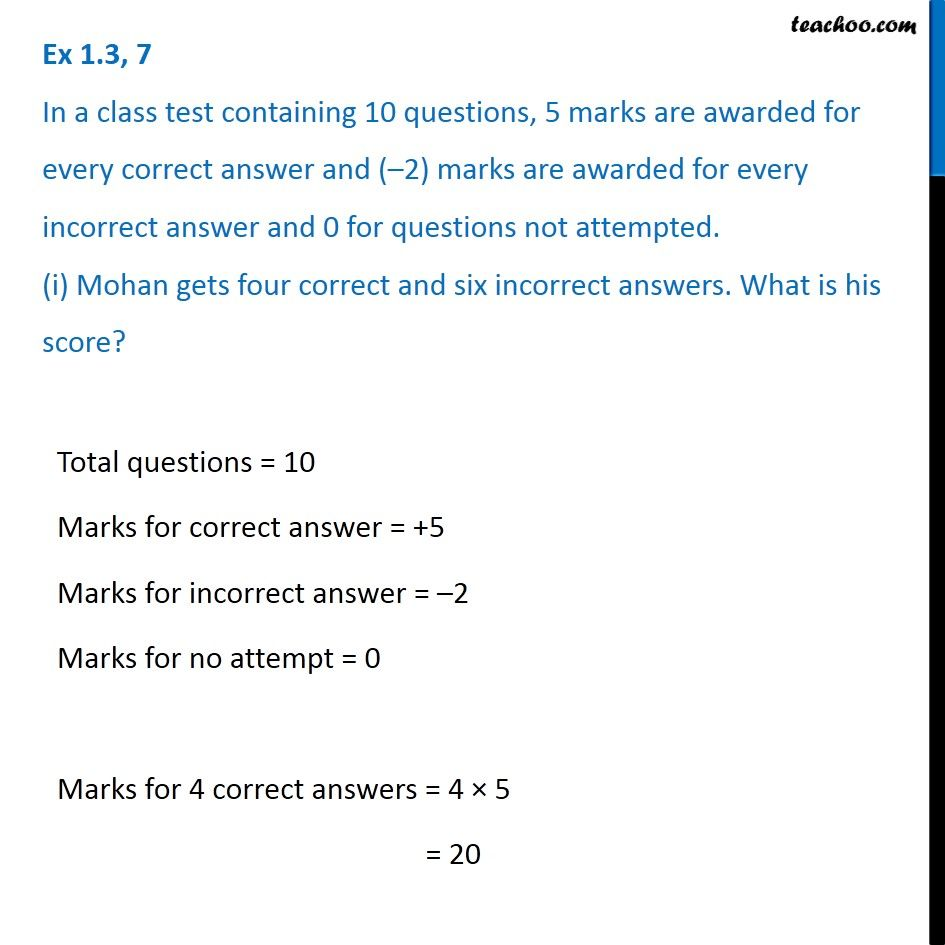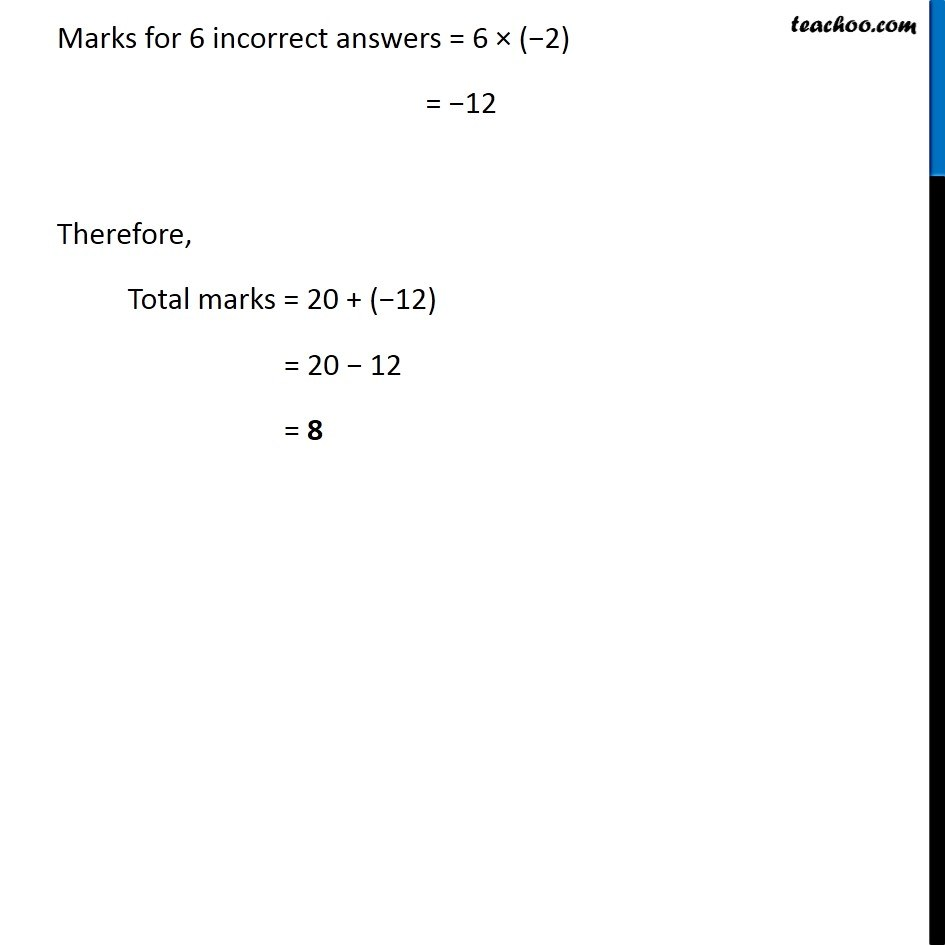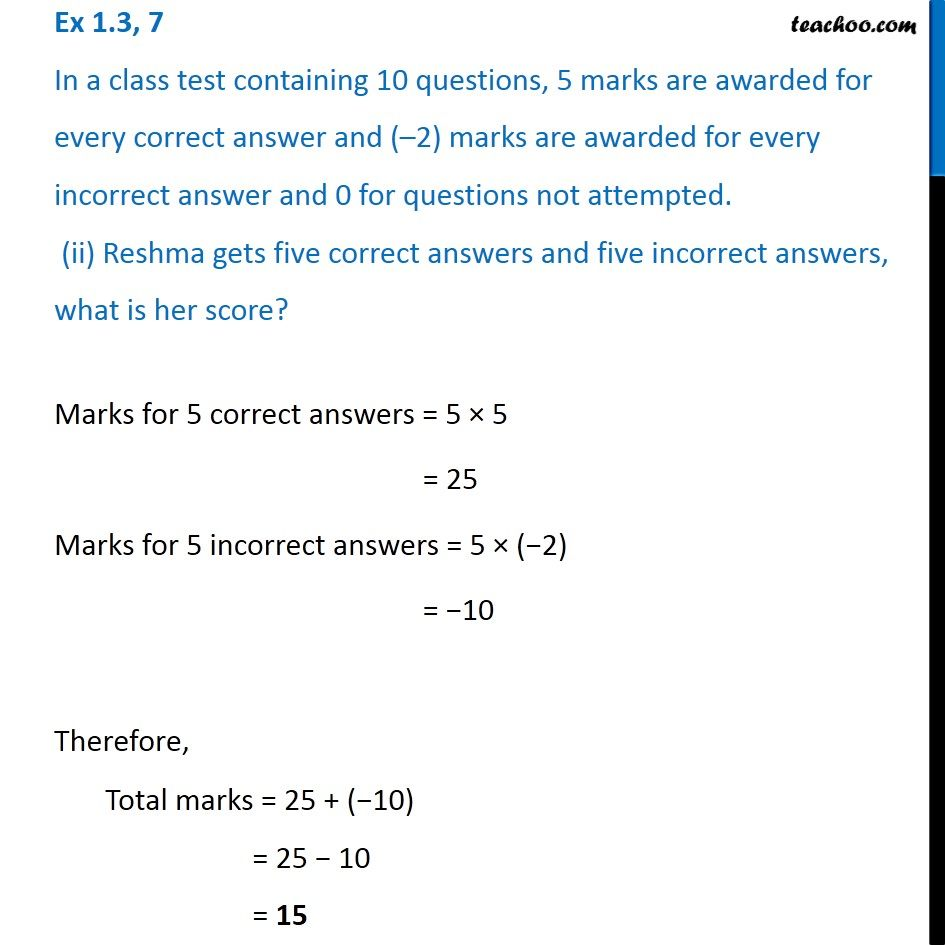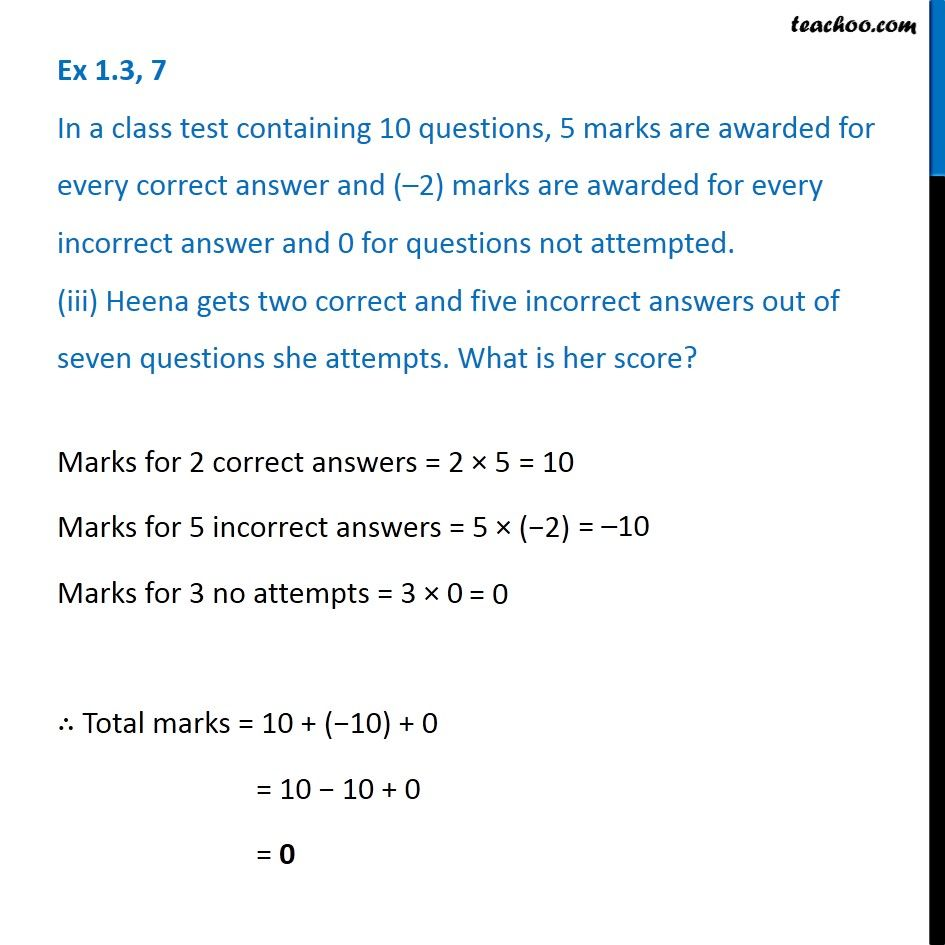1. Chapter 1 Class 7 Integers
2. Serial order wise
3. Ex 1.3

Transcript

Ex 1.3, 7 In a class test containing 10 questions, 5 marks are awarded for every correct answer and ( 2) marks are awarded for every incorrect answer and 0 for questions not attempted. (i) Mohan gets four correct and six incorrect answers. What is his score? Total questions = 10 Marks for correct answer = +5 Marks for incorrect answer = 2 Marks for no attempt = 0 Marks for 4 correct answers = 4 5 = 20 Marks for 6 incorrect answers = 6 ( 2) = 12 Therefore, Total marks = 20 + ( 12) = 20 12 = 8 Ex 1.3, 7 In a class test containing 10 questions, 5 marks are awarded for every correct answer and ( 2) marks are awarded for every incorrect answer and 0 for questions not attempted. (ii) Reshma gets five correct answers and five incorrect answers, what is her score? Marks for 5 correct answers = 5 5 = 25 Marks for 5 incorrect answers = 5 ( 2) = 10 Therefore, Total marks = 25 + ( 10) = 25 10 = 15 Ex 1.3, 7 In a class test containing 10 questions, 5 marks are awarded for every correct answer and ( 2) marks are awarded for every incorrect answer and 0 for questions not attempted. (iii) Heena gets two correct and five incorrect answers out of seven questions she attempts. What is her score? Marks for 2 correct answers = 2 5 Marks for 5 incorrect answers = 5 ( 2) Marks for 3 no attempts = 3 0 Total marks = 10 + ( 10) + 0 = 10 10 + 0 = 0

Ex 1.3

Chapter 1 Class 7 Integers
Serial order wise